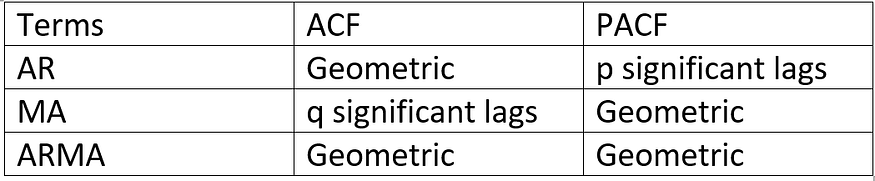2022-01-25
###### Interpreting ACF and PACF Plots for AR and MA models
2022-01-25

Selecting candidate Auto Regressive Moving Average (ARMA) models for time series analysis and forecasting, understanding Autocorrelation function (ACF), and Partial autocorrelation function (PACF) plots of the series are necessary to determine the order of AR and/ or MA terms. Though ACF and PACF do not directly dictate the order of the ARMA model, the plots can facilitate understanding the order and provide an idea of which model can be a good fit for the time-series data. In this article, primarily I share my experience in understanding the ACF, and PACF plots, and their significance in selecting the order of ARMA models.

ACF plot is a bar chart of coefficients of correlation between a time series and its lagged values. Simply stated: ACF explains how the present value of a given time series is correlated with the past (1-unit past, 2-unit past, …, n-unit past) values. In the ACF plot, the y-axis expresses the correlation coefficient whereas the x-axis mentions the number of lags. Assume that, y(t-1), y(t), y(t-1),….y(t-n) are values of a time series at time t, t-1,…,t-n, then the lag-1 value is the correlation coefficient between y(t) and y(t-1), lag-2 is the correlation coefficient between y(t) and y(t-2) and so on.

PACF is the partial autocorrelation function that explains the partial correlation between the series and lags itself. In simple terms, PACF can be explained using a linear regression where we predict y(t) from y(t-1), y(t-2), and y(t-3). In PACF, we correlate the “parts” of y(t) and y(t-3) that are not predicted by y(t-1) and y(t-2).

Identifying AR and MA orders by ACF and PACF plots:

Assume that, the time series is stationary, if not then we can perform transformation and/or differencing of the series to convert the series into a stationary process. Once the series is stabilized, we can plot the ACF and PACF plots to identify the orders of AR and MA terms in the ARMA model. At times, only AR terms or only MA terms are sufficient to model the process. Table 1 explains how to select AR and MA terms based on ACF and PACF :Table 1: AR and MA terms based on ACF and PACF

The ACF and PACF plots should be considered together to define the process. For the AR process, we expect that the ACF plot will gradually decrease and simultaneously the PACF should have a sharp drop after p significant lags. To define a MA process, we expect the opposite from the ACF and PACF plots, meaning that: the ACF should show a sharp drop after a certain q number of lags while PACF should show a geometric or gradual decreasing trend. On the other hand, if both ACF and PACF plots demonstrate a gradual decreasing pattern, then the ARMA process should be considered for modeling.

Fig. 1 and 2 illustrate ACF and PACF for a given stationary time series data. The ACF shows a gradually decreasing trend while the PACF cuts immediately after one lag. Thus, the graphs suggest that an AR (1) model would be appropriate for the time series.

Fig. 3 and 4 show ACF and PACF for a stationary time series, respectively. The ACF and PACF plots indicate that an MA (1) model would be appropriate for the time series because the ACF cuts after 1 lag while the PACF shows a slowly decreasing trend.

Fig. 5 & 6 show ACF and PACF for another stationary time series data. Both ACF and PACF show slow decay (gradual decrease). Hence, the ARMA (1,1) model would be appropriate for the series. Again, observing the ACF plot: it sharply drops after two significant lags which indicates that an MA (2) would be a good candidate model for the process. Therefore, we should experiment with both ARMA (1,1) and MA (2) for the process and later select the optimal model based on a performance metric like AIC (Akaike Information Criteria).